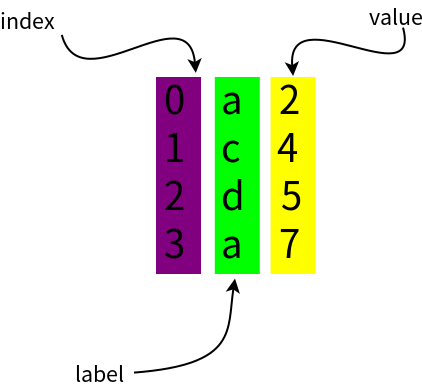# 2. Pandas的Series的创建

pandas的一维的数据结构Series有点像Python的列表list，可以存储一些数据，可以通过index访问Series里某位置上的数据，也可以通过标签label来访问某数据(这点有点类似于Python的字典dict)。 换句话说Series即可像list一样用index来标识Series里某数据，也可像dict那样通过标签来标识某数据。

## 2.1 创建Series的方法

• 不指定index创建series
``````import pandas as pd
a = pd.Series([2, 4, 5, 6])
print a
print a
print a[1 : 3]
``````

``````0    2
1    4
2    5
3    6
dtype: int64
2#a
1    4#a[1 : 3]
2    5
dtype: int64
``````

• 在Series里指定index 用户可以在pandas的Series构造函数里使用index属性，为新建的Series数据指定标识的标签label。
``````import pandas as pd
s = pd.Series([2, 4, 5, 6], index=list("abcd"))
print s
print "s[b]->", s["b"]
print 's[0 : 3]\n', s[0 : 3]
print 's["a" : "c"]\n', s["a" : "c"]
``````

``````a    2#print s
b    4
c    5
d    6
dtype: int64
s[b]-> 4
s[0 : 3]
a    2
b    4
c    5
dtype: int64
s["a" : "c"]
a    2
b    4
c    5
dtype: int64
``````
• 通过字典构造 在Series构造函数里传入字典数据，即提供了数据又提供了index或者label。
``````import pandas as pd
s = pd.Series({"a" : 100, "b" : 200, "e" : 300})
print s
``````

``````a    100# print s
b    200
e    300
dtype: int64
``````

## 2.2 常用创建Series的方法

``````import pandas as pd
s = pd.Series({"a" : 100, "b" : 200, "e" : 300})
print s
i = ["a", "c", "d", "a"]
v = [2, 4, 5, 7]
t = pd.Series(v, index=i, name = "col_name")
print t
``````

``````a    100# print s
b    200
e    300
dtype: int64
a    2# print t
c    4
d    5
a    7
Name: col_name, dtype: int64
``````

Series构造函数的name参数是给这列数据指定字段名。从结果可以看出t有两个名为'a'的label，值分别为2和7。``````import pandas as pd
s = pd.Series({"a" : 100, "b" : 200, "e" : 300})
print s
i = ["a", "c", "d", "a"]
v = [2, 4, 5, 7]
t = pd.Series(v, index=i, name = "col_name")
print t
print "t['a']\n", t["a"]
print 't', t
print 't', t
``````

``````a    100# print s
b    200
e    300
dtype: int64
a    2# print t
c    4
d    5
a    7
Name: col_name, dtype: int64
t['a']
a    2
a    7
Name: col_name, dtype: int64
t 2
t 7
``````